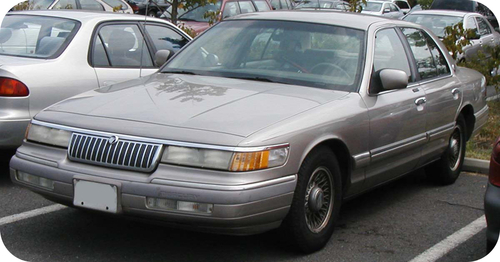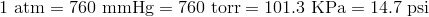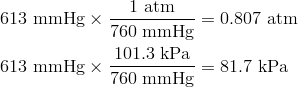Pressure Units and Conversions

Learning Objectives

• Be able to convert between different units of pressure.

ExamplesCan you guess how old this car is?

There are several benefits to maintaining the proper air pressure in a car tire. The ride is smoother and safer than with lowered pressure. The car gets better gas mileage and the tires don’t wear out as fast. The recommended pressure for that model of car (usually somewhere between 32-35 psi) is usually listed in the owner’s manual or stamped somewhere inside the door. The pressure on the tire is the maximum pressure for that tire, not the recommended one. Tire pressure is best measured when the tire is cold since driving the car for a while will heat up the air in the tire and increase the pressure.

Pressure Units and Conversion

A barometer measures gas pressure by the height of the column of mercury. One unit of gas pressure is the millimeter of mercury (mmHg). An equivalent unit to the mmHg is called the torr, in honor of the inventor of the barometer, Evangelista Torricelli. The pascal (Pa) is the standard unit of pressure. A pascal is a very small amount of pressure, so the more useful unit for everyday gas pressures is the kilopascal (kPa). A kilopascal is equal to 1000 pascals. Another commonly used unit of pressure is the atmosphere (atm). Standard atmospheric pressure is called 1 atm of pressure and is equal to 760 mmHg and 101.3 kPa. Atmospheric pressure is also often stated as pounds/square inch (psi). The atmospheric pressure at sea level is 14.7 psi.It is important to be able to convert between different units of pressure. To do so, we will use the equivalent standard pressures shown above.

Sample Problem: Pressure Unit Conversions

The atmospheric pressure in a mountainous location is measured to be 613 mmHg. What is this pressure in atm and in kPa?

Step 1: List the known quantities and plan the problem.

Known

• given: 613 mmHg
• 1 atm = 760 mmHg
• 101.3 kPa = 760 mmHg

Unknown

• pressure = ? atm
• pressure = ? kPa

Use conversion factors from the equivalent pressure units to convert from mmHg to atm and from mmHg to kPa.

Step 2: Solve.The air pressure is about 80% that of standard atmospheric pressure at sea level. For significant figure purposes, the standard pressure of 760 mmHg has three significant figures.

Key Takeaways

Summary

• Calculations are described for converting between different pressure units.

Exercises

Practice

Use the link below to practice conversion calculations:

Key Takeaways

Review

Questions

1. Who invented the barometer?
2. One atm = ___ torr?
3. 14.7 psi = ___ kPa
4. 760 mm = ___ psi?
5. The pressure in a car tire is 35 psi. How many atmospheres is that?

Glossary

• atmosphere: Common unit of pressure. Abbreviated as “atm.”
• pascal: The standard unit of pressure. Abbreviated as “Pa.”